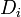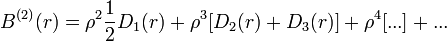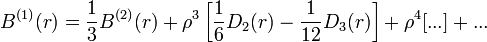2022: SklogWiki celebrates 15 years on-line

# Difference between revisions of "Bridge function"

The bridge functions are infinite series of irreducible diagrams$D_i$:$B^{(2)}(r) = \rho^2 \frac{1}{2} D_1(r) + \rho^3 [D_2(r) + D_3(r)] + \rho^4[...]+...$

and$B^{(1)}(r) = \frac{1}{3} B^{(2)}(r) + \rho^3 \left[\frac{1}{6}D_2(r) - \frac{1}{12}D_3(r)\right] + \rho^4[...]+...$

where$\rho$ is the density of the fluid.

## Universality of the Bridge functional

For Rosenfeld's principle of the universality of the bridge functional see .